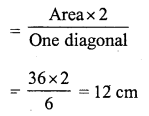# RS Aggarwal Class 7 Solutions Chapter 20 Mensuration Ex 20G

In this chapter, we provide RS Aggarwal Solutions for Class 7 Chapter 20 Mensuration Ex 20G for English medium students, Which will very helpful for every student in their exams. Students can download the latest RS Aggarwal Solutions for Class 7 Chapter 20 Mensuration Ex 20G Maths pdf, free RS Aggarwal Solutions Class 7 Chapter 20 Mensuration Ex 20G Maths book pdf download. Now you will get step by step solution to each question.

### RS Aggarwal Solutions for Class 7 Chapter 20 Mensuration Ex 20G Download PDF

Question 1.
Solution:
(c)
Length of rectangle AB = 16 cm
and diagonal BD = 20 cmBut, in right ∆ABD
BD² = AB² + AD²
⇒ (20)² = (16)² + AD²
⇒ 400 = 256 + AD²
⇒ AD² = 400 – 256 = 144 = (12)²
⇒ AD = 12 cm
Area = l x b = 16 x 12 = 192 cm²

Question 2.
Solution:
(b)
Diagonal of square = 12 cm
Let side = 9
diagonal = √2 aQuestion 3.
Solution:
(b)
Area = 200 cm²
side = √200 =√2 x 10
and diagonal = √2 a = √2 x √2 x 10 = 20

Question 4.
Solution:

(a)
Area of square = 0.5 hectare = 0.5 x 10000 = 5000 m²
= √10000 = 100 m

Question 5.
Solution:
(c)
Perimeter of rectangle = 240m
l + b = 2402 = 120 m
Let breadth = x, then length = 3x .
3x + x = 120
⇒ 4x = 120
⇒ x = 30
Length = 3x = 3 x 30 = 90 m

Question 6.
Solution:
Let original side of square = x
area = x²Question 7.
Solution:
(b)
Let side of square = a
Then its diagonal = √2 a
Now, area of square = a²
and area of square on diagonal = (√2 a)² = 2a²
Ratio = a² : 2a² = 1 : 2

Question 8.
Solution:
(c)
If perimeters of a square and a rectangle are equal Then the area of the square will be greater than that of a rectangle
A > B

Question 9.
Solution:
(b)
Perimeter of rectangle = 480mQuestion 10.
Solution:
(a)
Total cost of carpet = Rs. 6000
Rate per metre = Rs. 50Question 11.
Solution:
(a)
Sides are 13 cm, 14 cm, 15 cmQuestion 12.
Solution:
(b)
Base of triangle = 12 m
and height = 8m
Area= 12 x Base x height
= 12 x 12 x 8 = 48 m²

Question 13.
Solution:
(b)
Let side = a
then area = √34 a²Question 14.
Solution:
(c)
Side of an equilateral triangle = 8cmQuestion 15.
Solution:
(b)
Let a be the side of an equilateral triangleQuestion 16.
Solution:
(b)
One side (Base) of parallelogram = 16 cm
and altitude = 4.5 cm
Area = base x altitude = 16 x 4.5 = 72 cm²

Question 17.
Solution:
(b)
Length of diagonals of a rhombus are 24 cm and 18 cmQuestion 18.
Solution:
(c)
Let r be the radius of the circle Then
c = 2πr
2πr – r = 37Question 19.
Solution:
(c) Perimeter of room = 18 m
and height = 3 m
Area of 4 walls = Perimeter x height = 18 x 3 = 54 m²

Question 20.
Solution:
(a) Area of floor = l x b = 14 x 9 = 126 m²
Area of carpet = 126 m²Question 21.
Solution:
(c)
Perimeter = 46 cmQuestion 22.
Solution:
(b)
Ratio in area of two squares = 9 : 1
Let area of bigger square = 9x²
and of smaller square = x²
Side of bigger square = √9x² = 3x
and perimeter = 4 x side = 4 x 3x = 12x
Side of smaller square = √x² = x
Perimeter = 4x
Now ratio in their perimeter = 12x : 4x = 3 : 1

Question 23.
Solution:
(d)
Let the diagonals of two square be 2d and d
Area of bigger square 2 (2d)² = 8d²
and of smaller = 2 (d)² = 2d²
Ratio in their area = 8d22d2=41
= 4 : 1

Question 24.
Solution:
(c)
Side of square = 84 m
Area of square = (84)² = 7056 m²
Area of rectangle = 7056 m²
Length of rectangle = 144 mQuestion 25.
Solution:
(d)
Side of a square = a
Area = a²
Side of equilateral triangle = aQuestion 26.
Solution:
(a)
Let a be the side of a square
Area = a²
Then area of circle = a²
Let r be the radiusQuestion 27.
Solution:
(b)
Let each side of an equilateral triangle = a
Then area = √34 a²
Now radius of the circle = a
Then area = πr² = πa²Question 28.
Solution:
(c)
Area of rhombus = 36 cm²
Length of one diagonal = 6 cm
Length of second diagonalQuestion 29.
Solution:
(d)
Area of a rhombus =144 cm²
Let one diagonal (d1) = a
then Second diagonal (d2) = 2aLarger diagonal = 2a = 2 x 12 = 24 cm

Question 30.
Solution:
(c)
Area of a circle = 24.64 m²Question 31.
Solution:
(c)
Let original radius = r
Then its area = πr²
Radius of increased circle = r + 12r = 7 – 1 = 6
⇒ r = 62 = 3
Radius of original circle = 3 cm

Question 32.
Solution:
(c)
Radius of a circular wheel (r) = 1.75 mAll Chapter RS Aggarwal Solutions For Class 7 Maths

—————————————————————————–

All Subject NCERT Exemplar Problems Solutions For Class 7

All Subject NCERT Solutions For Class 7

*************************************************

I think you got complete solutions for this chapter. If You have any queries regarding this chapter, please comment on the below section our subject teacher will answer you. We tried our best to give complete solutions so you got good marks in your exam.

If these solutions have helped you, you can also share rsaggarwalsolutions.in to your friends.